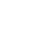Choose language# Magical Power of Quantum Mechanics

News & EventsServices & Solutions

News & Events

Career

Magical Power of Quantum MechanicsEvents

News

Magical Power of Quantum Mechanics

## Application of Electrostatic Potential Map in Acidity Estimation

### QM Magic Class | Chapter 9

In Chapter 8, we discussed modeling of chemical reactions, calculation of the activation energy of reaction and structure of transition state, etc. In this article, we will discuss how these routine calculations are used to guide our synthetic planning.

Let’s start with two practical questions for the following alkylation of pyrazole 1 (Figure 1). Will the alkylation be selective on N-1 or N-2? If it is not selective, will there be a reliable way to estimate the product ratio?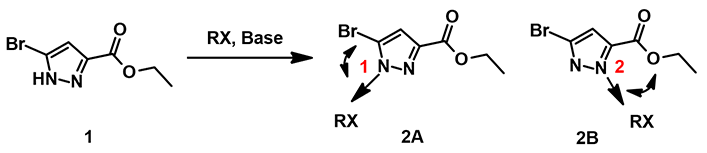Figure 1. Regioselectivity of N-alkylation on pyrazole 1

First, we calculate for the Electrostatic Potential Map (Chapter 3) of the two tautomeric forms of pyrazole 1 to estimate the relative acidity of the NH group (Figure 2, A and B). The electrostatic potential of the NH group in tautomer A (281 kJ/mol) is found to be slightly higher than that in tautomer B (266 kJ/mol). However this difference may not be consequential, since after deprotonation, charge is delocalized within the pyrazole molecule. Indeed, HOMO calculated for the resultant pyrazole anion shows similar lobe size on N-1 and N-2 (Figure 2, C). These couldn’t help us to differentiate the two potential alkylation pathways.

### Reaction Energy Profile

QM calculation enables us to model stepwisely the bond forming & breaking process in a chemical reaction, establish the transition state structures, estimate the associated activation energy, compare the relative difference of competing paths, and calculate for the potential product ratio.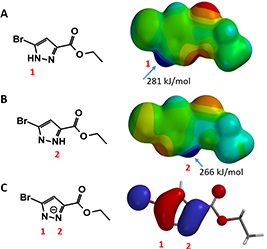Figure 2. A and B: Electrostatic Potential Maps of the two tautomeric form of pyrazole 1. C: HOMO of pyrazole 1 anion

### Establish the Reaction Model

For the pyrazole alkylation in consideration, we assume a SN2 mechanism, with pyrazole N gradually approaches the alkyl halide, forming the C-N bond and breaking the C-X bond in a concerted manner.

We set up two calculations, using CH3Br as our generic alkylating reagent, one approaches N1 of pyrazole 1, and the other interacts with N2, starting with a C-N distance of 3.8 Å, and calculate for the system energy with a step size of 0.1 Å (Figure 3).

Energy of the system starts to rise when the distance between C-N atoms is shortened to 3.0 Å, indicating that the reactant molecules start to interact. When the bond length is shortened to about 2.1 Å, the energy level reaches the peak, reaction reaches the transition state. The energy difference between these two points is our estimated activation energy.

### Estimation of Product Ratio

Activation energy calculated for N-1 and N-2 alkylation is 10.77 and 9.24 kcal/mol, respectively (Figure 3), suggesting that the reaction will occur more readily at N-2.Figure 3. Reaction energy profiles of N1 (red), N2 (blue) alkylation paths

Plugging into Arrhenius Equation the estimated difference in activation energies (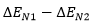, 1.53 kcal/mol), an estimated product ratio of ~13:1 at 25 ºC is obtained.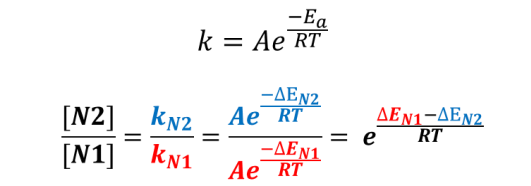Figure 4. Calculating product ratio with Arrhenius equation

### Relative Difference in Activation Energy Controls Product Ratio

Table 1 illustrates how changes in relative difference in activation energy (ΔE1-ΔE2) and reaction temperature affect product ratio, an important consideration in our synthetic planning and execution.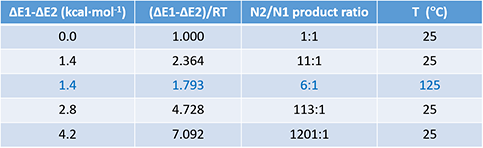Table 1. Product ratio obtained with different ΔΔE and temperature

For a reaction with two competing pathways, with a difference of 1.4 kcal/mol in activation energy, the product ratio will be 11:1 with reaction run at 25 ºC; the ratio will decrease to 6:1 when the reaction temperature is raised to 125 ºC.

We found in practice that when the activation energy difference is around 1.4 kcal/mol, we can empirically adjust reaction temperature and solvent to optimize the ratio. On the other hand, if the calculated activation energy difference is above 3 kcal/mol, it is difficult to reverse the intrinsic bias.

In summary, through QM calculations of competing alkylation paths of pyrazole, we could calculate the difference in their activation energies, estimate the ratio of the products, and use the result to guide our synthetic planning and execution.

### Building on What We Just Learned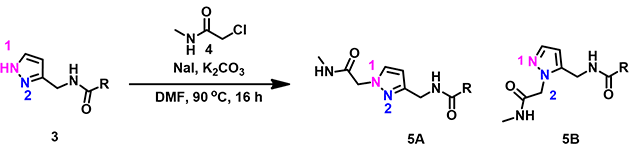Alkylation of pyrazole 3 with simple alkyl halide is N-1 selective. However, reaction with chloroacetamide 4 provides only N-2 alkylation product. What could have possibly caused the reversal in selectivity?

References:

 W. Hehre and S. Ohlinger, A Guide to Molecular Mechanics and Quantum Chemical Calculations.Irvine, CA, USA: Wavefunction, Inc., 2003.

 Spartan’18 Tutorial and User’s Guide. Irvine, CA, USA: Wavefunction, Inc., 2019; pp 116-118.

 S. Liu & L.G. Pedersen, J. Phys. Chem. A. 2009, 113, 3648.

 无机化学 第四版 （杨宏孝 王建辉 著） 高等教育出版社第七章 270-279 页。